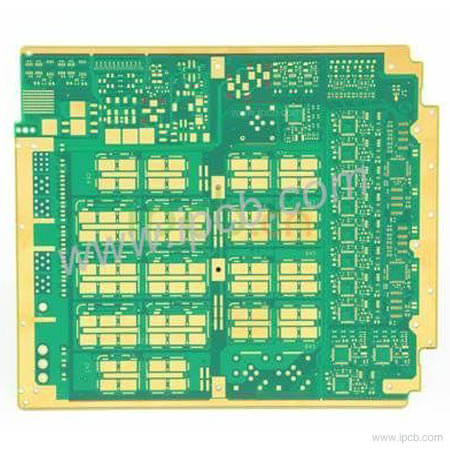전문 고주파 회로 기 판,고속 회로 기 판,IC 패 키 징 기 판,반도체 테스트 판,HDI 회로 기 판,소프트 하 드 결합 판,양면 다 층 판,PCB 디자인 및 PCBA 제조

### 빠 른 가격 획득.

sales@ipcb.com# IC Test Board## IC Test Board

•18Layers Communication Base Station PCB

Model : 18Layers Communication Base Station PCB

Material : panasonic M6

Layer : 18Layers

Copper Thickness : 0.5-1OZ

Finished Thickness : 2.0mm

Surface Treatment : Immersion Gold

Trace/Space : 4mil/4mil(0.1MM/0.1MM)

Min Hole : 0.2mm(8mil)

Special process : Metal edging

Application : communication base station PCB

Product details Technical specification

1. The commonly used PCB medium is FR4, and the dielectric constant relative to air is 4.2-4.7. The dielectric constant varies with temperature, and the maximum variation range can reach 20% in the temperature range of 0-70 ℃. The change of dielectric constant will lead to 10% change of line delay. The higher the temperature, the greater the delay. The higher the frequency, the smaller the dielectric constant. The capacitance and time delay can be calculated by 4.5 below 100m.

2. The transmission speed of the inner layer signal in the general FR4 PCB is 180ps / inch (1inch = 1000mil = 2.54cm). The surface layer generally depends on the situation, generally between 140 and 170.

3. The actual capacitance can be simply equivalent to L, R, C series connection. The capacitor has a resonance point, which will be perceptual at high frequency (beyond this resonance point). The resonance point will be different if the capacitance value and process of capacitor are different, and the products produced by different PCB manufacturers will also be very different. The resonant point mainly depends on the equivalent series inductance. Now, for example, for a 100nF chip capacitor, the equivalent series inductance is about 0.5nh, and the ESR (equivalent series resistance) value is 0.1 Ω, then the filtering effect is the best when it is about 24m, and the AC impedance is 0.1 Ω. The equivalent inductance of a 1NF chip capacitor is 0.5nh (the difference between different capacitance values is not too big), and the ESR is 0.01 Ω, which will have the best filtering effect around 200m. In order to achieve a better filtering effect, we use different capacitance value of the capacitor combination. However, due to the effect of equivalent series inductance and capacitance, there will be a resonance point between 24m and 200m, at which the maximum impedance is greater than that of a single capacitor. It's a result we don't want. (from 24m to 200m, small capacitance is capacitive and large capacitance is inductive. The parallel connection of two capacitors is equivalent to LC parallel connection. The sum of ESR values of the two capacitors is the series resistance of the LC circuit. If LC is parallel, if the series resistance is 0, then there will be an infinite impedance at the resonance point, which has the worst filtering effect. On the contrary, the series resistance will suppress the parallel resonance phenomenon, thus reducing the impedance of LC resonator at the resonance point. To mitigate this effect, larger ESR capacitors can be used as appropriate. ESR is equivalent to the series resistance in the resonant network, which can reduce the Q value and make the frequency characteristics flat. Increasing ESR will make the whole impedance consistent. The impedance will increase in the frequency band lower than 24m and higher than 200m, while it will decrease in the frequency band of 24m and 200m. Therefore, the frequency band of board switching noise should be considered comprehensively. In some foreign designs, when large and small capacitors are connected in parallel, a few ohm resistors are connected in series on small capacitors (680pf), which is probably due to this consideration. (from the above parameters, the capacitance Q value of 1NF is 10 times of that of 100nF. Since there is no specific equivalent string sense and ESR value from the manufacturer, the parameters of the above example are inferred based on the data seen in the past. But the deviation should not be too large. In the past, the resonant frequencies of 1NF and 100nF ceramic capacitor are 100m and 10m respectively. Considering the chip capacitor L is much smaller, and no reliable value is found.

We will respond very quickly.

Model : 18Layers Communication Base Station PCB

Material : panasonic M6

Layer : 18Layers

Copper Thickness : 0.5-1OZ

Finished Thickness : 2.0mm

Surface Treatment : Immersion Gold

Trace/Space : 4mil/4mil(0.1MM/0.1MM)

Min Hole : 0.2mm(8mil)

Special process : Metal edging

Application : communication base station PCB